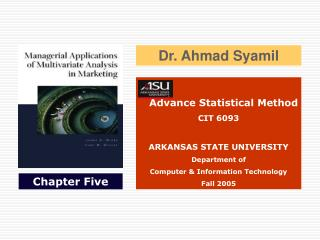DownloadDownload PresentationAdvance Statistical Method CIT 6093 ARKANSAS STATE UNIVERSITY Department of Computer & Information Technology Fall

Advance Statistical Method CIT 6093 ARKANSAS STATE UNIVERSITY Department of Computer & Information Technology Fall

Télécharger la présentationAdvance Statistical Method CIT 6093 ARKANSAS STATE UNIVERSITY Department of Computer & Information Technology Fall

- - - - - - - - - - - - - - - - - - - - - - - - - - - E N D - - - - - - - - - - - - - - - - - - - - - - - - - - -
Presentation Transcript

1. Dr. Ahmad Syamil Advance Statistical Method CIT 6093 ARKANSAS STATE UNIVERSITY Department of Computer & Information Technology Fall 2005 Chapter Five

2. OBJECTIVES • APPLICATION OF MULTIPLE CORRELATION/REGRESSION ANALYSIS • MULTIPLE CORRELATION • MULTIPLE REGRESSION • TECHNICAL DESCRIPTION • COMMON PROBLEMS IN MULTIPLE CORRELATION/REGRESSION ANALYSIS

3. APPLICATION OF MULTIPLE CORRELATION/REGRESSION ANALYSIS Multiple Correlation • Statistical Techniques for Measuring the Closeness of the Relationship Between Variables • It Measures the Degree to which Changes in One Variable are Associated with Changes in Another • It can Only Indicate the Degree of Association or Covariance Between Variables. Covariance is a Measure of the Extent to which Two Variables are Related Cont’d…

4. APPLICATION OF MULTIPLE CORRELATION/REGRESSION ANALYSIS Multiple Regression • Requires Two Operations • Derive an Equation, Called the Regression Equation, and a Line Representing the Equation to Describe the Shape of the Relationship Between the Variables. • Estimate the Dependent Variable (Y) from the Independent Variable (X), Based on the Relationship Described by the Regression Equation.

5. COMMON PROBLEMS IN MULTIPLE CORRELATION/REGRESSION ANALYSIS Technical Description • Graphical Representations • Statistical Significance of R • Interpretation of Multiple Correlation Coefficients • Interpretation of Multiple Regression Coefficients • Requirement/Assumptions for Multiple Regression Cont’d…

6. COMMON PROBLEMS IN MULTIPLE CORRELATION/REGRESSION ANALYSIS Missing Values: How to Deal the Issue? • Eliminate a Respondent or Company Entirely • Provide an Estimated Value Cont’d…

7. COMMON PROBLEMS IN MULTIPLE CORRELATION/REGRESSION ANALYSIS Uniform Ratings A Related Question is what to do About Respondents Who Have no Missing Values But Give the Same Rating for Nearly All Product Attributes, Attitudes, or Important Ratings. Cont’d…

8. COMMON PROBLEMS IN MULTIPLE CORRELATION/REGRESSION ANALYSIS • Multi-Collinearity • High correlation exists between two independent variables • This means the two variables contribute redundant information to the multiple regression model Cont’d…

9. COMMON PROBLEMS IN MULTIPLE CORRELATION/REGRESSION ANALYSIS • Dummy Variables • Categorical Explanatory Variable with Two or More Levels… • Yes or No, On or Off, Male or Female • Code as 0 or 1 • Regression Intercepts are Different if the Variable is Significant • Assume Equal Slopes for Other Variable